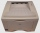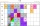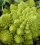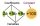# Fraction calculator

The calculator performs basic and advanced operations with fractions, expressions with fractions combined with integers, decimals, and mixed numbers. It also shows detailed step-by-step information about the fraction calculation procedure. Solve problems with two, three, or more fractions and numbers in one expression.

## Result:

### 2/3^3 = 8/27 = 0.2962963

Spelled result in words is eight twenty-sevenths.

### How do you solve fractions step by step?

1. Exponentiation: 2/3 ^ 3 = 23/33 = 8/27

In words - two thirds raised to the power of cubed = eight twenty-sevenths.

#### Rules for expressions with fractions:

Fractions - use the slash “/” between the numerator and denominator, i.e., for five-hundredths, enter 5/100. If you are using mixed numbers, be sure to leave a single space between the whole and fraction part.
The slash separates the numerator (number above a fraction line) and denominator (number below).

Mixed numerals (mixed fractions or mixed numbers) write as non-zero integer separated by one space and fraction i.e., 1 2/3 (having the same sign). An example of a negative mixed fraction: -5 1/2.
Because slash is both signs for fraction line and division, we recommended use colon (:) as the operator of division fractions i.e., 1/2 : 3.

Decimals (decimal numbers) enter with a decimal point . and they are automatically converted to fractions - i.e. 1.45.

The colon : and slash / is the symbol of division. Can be used to divide mixed numbers 1 2/3 : 4 3/8 or can be used for write complex fractions i.e. 1/2 : 1/3.
An asterisk * or × is the symbol for multiplication.
Plus + is addition, minus sign - is subtraction and ()[] is mathematical parentheses.
The exponentiation/power symbol is ^ - for example: (7/8-4/5)^2 = (7/8-4/5)2

#### Examples:

subtracting fractions: 2/3 - 1/2
multiplying fractions: 7/8 * 3/9
dividing Fractions: 1/2 : 3/4
exponentiation of fraction: 3/5^3
fractional exponents: 16 ^ 1/2
adding fractions and mixed numbers: 8/5 + 6 2/7
dividing integer and fraction: 5 ÷ 1/2
complex fractions: 5/8 : 2 2/3
decimal to fraction: 0.625
Fraction to Decimal: 1/4
Fraction to Percent: 1/8 %
comparing fractions: 1/4 2/3
multiplying a fraction by a whole number: 6 * 3/4
square root of a fraction: sqrt(1/16)
reducing or simplifying the fraction (simplification) - dividing the numerator and denominator of a fraction by the same non-zero number - equivalent fraction: 4/22
expression with brackets: 1/3 * (1/2 - 3 3/8)
compound fraction: 3/4 of 5/7
fractions multiple: 2/3 of 3/5
divide to find the quotient: 3/5 ÷ 2/3

The calculator follows well-known rules for order of operations. The most common mnemonics for remembering this order of operations are:
PEMDAS - Parentheses, Exponents, Multiplication, Division, Addition, Subtraction.
BEDMAS - Brackets, Exponents, Division, Multiplication, Addition, Subtraction
BODMAS - Brackets, Of or Order, Division, Multiplication, Addition, Subtraction.
GEMDAS - Grouping Symbols - brackets (){}, Exponents, Multiplication, Division, Addition, Subtraction.
Be careful, always do multiplication and division before addition and subtraction. Some operators (+ and -) and (* and /) has the same priority and then must evaluate from left to right.

## Fractions in word problems:

• Two xeroxesThe performances of the two copiers are in the ratio 3: 4. A machine with higher power will make 7,200 copies in one hour. How many copies will both machines make together in 5 hours?
• Three machinesThe power of the three machines is 2: 3: 5. Two most powerful machines produce 400 parts per hour. How many components make all three machines in 3 hours?
• Fraction unknownsDivide of fractions with unknowns: Fraction 1: The quantity x squared plus 6 times x plus 9 over the quantity x minus 1. Fraction 2: the quantity x squared minus 9 over the quantity x squared minus 2 times x plus 1.  Find Fraction 1 over Fraction 2.
• MohammedMohammed grew a garden last year. His plot of land was 6 3/4 feet by 10 feet. He wants to have twice as much area and his garden this year how much area will the new garden take up?
• A tileA tile setter is covering 5ft by 5ft square shower wall. Each tile covers 4 5/8in by 4 5/8in square. How many rows of tile are needed to reach 5ft? How many tiles are needed to cover 5ft by 5ft square
• BMI indexCalculate BMI (body mass index, an index indicating obesity, overweight, normal weight, underweight) man weighing m = 71 kg and height h = 170 cm. The index is calculated according to equation (formula): BMI = (m)/(h2) With BMI index is possible to compa
• SeawaterSeawater has a density of 1025 kg/m3, ice 920 kg/m3. 8 liters of seawater froze and created a cube. Calculate the size of the cube edge.
• Simplest formFind the simplest form of the following expression: 3 to the 2nd power - 1/4 to the 2nd power.